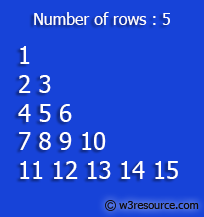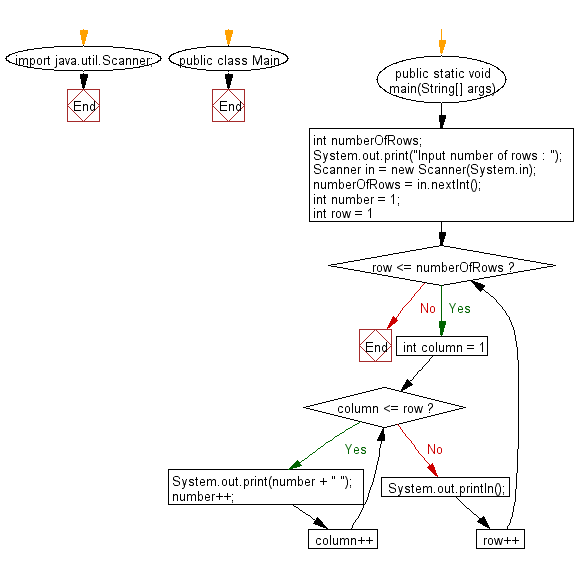﻿ Java - Print the Floyds Triangle

# Java: Print the Floyd's Triangle

## Java Conditional Statement: Exercise-20 with Solution

Write a Java program to print Floyd's Triangle.

Floyd's triangle is a right-angled triangular array of natural numbers, used in computer science education. It is named after Robert Floyd. It is defined by filling the rows of the triangle with consecutive numbers, starting with a 1 in the top left corner:

Test Data
Input number of rows : 5

Pictorial Presentation:Sample Solution:

Java Code:

``````import java.util.Scanner;
public class Main {
public static void main(String[] args)
{
int numberOfRows;
System.out.print("Input number of rows : ");
Scanner in = new Scanner(System.in);
numberOfRows = in.nextInt();
int number = 1;
for (int row = 1; row <= numberOfRows; row++)
{
for (int column = 1; column <= row; column++)
{
System.out.print(number + " ");
number++;
}
System.out.println();
}
}
}
```
```

Sample Output:

```Input number of rows :  5
1
2 3
4 5 6
7 8 9 10
11 12 13 14 15
```

Flowchart:Java Code Editor:

What is the difficulty level of this exercise?

Test your Programming skills with w3resource's quiz.

﻿

## Java: Tips of the Day

IsPowerOfTwo

Checks if a value is positive power of two.

To understand how it works let's assume we made a call IsPowerOfTwo(4).

As value is greater than 0, so right side of the && operator will be evaluated.

The result of (~value + 1) is equal to value itself. ~100 + 001 => 011 + 001 => 100. This is equal to value.

The result of (value & value) is value. 100 & 100 => 100.

This will value the expression to true as value is equal to value.

```public static boolean isPowerOfTwo(final int value) {
return value > 0 && ((value & (~value + 1)) == value);
}
```

Ref: https://bit.ly/3sA5d4I

We are closing our Disqus commenting system for some maintenanace issues. You may write to us at reach[at]yahoo[dot]com or visit us at Facebook